Reach Us+44-1522-440391
Fault Diagnosis of Spinning Industrial Systems by Using Wavelet Analysis and Soft Computer Classifier | OMICS International
Industrial Engineering & Management

# Fault Diagnosis of Spinning Industrial Systems by Using Wavelet Analysis and Soft Computer Classifier

Ahmed E. Amin Mohamed*

Department of Computer Science, Mansoura University, Mansoura 35516, Egypt

*Corresponding Author:
Ahmed E. Amin Mohamed
Department of Computer Science
Mansoura University
Mansoura 35516, Egypt
E-mail: [email protected]

Received January 01, 2013; Accepted February 28, 2013; Published March 04, 2013

Citation: Mohamed AEA (2013) Fault Diagnosis of Spinning Industrial Systems by Using Wavelet Analysis and Soft Computer Classifier. Ind Eng Manage 2:106. doi:10.4172/2169-0316.1000106

Copyright: © 2013 Mohamed AEA. This is an open-access article distributed under the terms of the Creative Commons Attribution License, which permits unrestricted use, distribution, and reproduction in any medium, provided the original author and source are credited.

Visit for more related articles at Industrial Engineering & Management

#### Abstract

Any industry needs an efficient predictive plan in order to optimize the production quality and improve the economy of the plant by reducing the defective product and produce final product fits its end-use.
In the textile industry, mass variation is an important property of yarn and is generally described by the diagram and spectrogram. The main parameter used for characterizing yarn evenness is the Coefficient of Variation (C.V %).
This parameter is based on the “cut and weight” method. Practice in the industry test length of 8 mm was used. This means that the C.V% corresponds to an electronic cut length of 8 millimeter. A diagram of the mass variation can also be produced to provide an overall profile of yarn irregularity.
For spun yarns, the evenness tester produces a spectrogram that covers a range of wavelengths from 2 cm to 1280 m. It assesses periodic mass variations, which occur at least 25 times as being statistically significant. Periodic variations are typically caused by mechanical defects (e.g. drafting faults).
This paper presented a new method based on multi-resolution analysis, for the classification of yarn mechanical defects diagnosis. It is constituted of two stages architecture: in the first stage a set of features are extracted from yarn signal by a wavelet analysis. The second stage is devoted to the classification of defect from the features by using Probabilistic Neural Network (PNN). Naïve Bayes algorithm and Bayes net algorithm is taken for classification and compared. The novelty of the proposed method resides in the ability not only with higher precision, but also with dimensionality reduction and higher speed than method of Fourier transform and mathematical statistics.

#### Keywords

Fault diagnosis; Wavelet; Neural network

#### Introduction

Classification is the field devoted to the study of methods designed to categorize data into distinct classes. This categorization can be divided to distinct labeling of the data (supervised learning), division of the data into classes (unsupervised learning), selection of the most significant features of the data (feature selection), or a combination of more than one of these tasks.

Generally, In textile industry, textiles are inspected manually for defects, but some problems arise in this visual inspection, such as excessive time consumed, human subjective factors, stress on mind and body, and fatigue. These problems further influence production volume and inspection accuracy. Therefore, techniques that can replace manual inspection have emerged .

In recent years, neural networks have been used to inspect yarn, fabric and cloth defects and to identify their types . Neural networks are among the best classifier used for fault detection due to their nonparametric nature and ability to describe complex decision regions.

Usually, in ANN, the available data are divided into three groups. The first group is the training set. The second group is the validation set, which is useful when the network begins to over-fit the data so the error on the validation set typically begins to rise; during this time the training is stopped for a specified number of iterations (max fails) and the weights and biases at the minimum of the validation error are returned. The last group is the performance test set, which is useful to plot the test set error during the training process .

A key issue in many neural network applications is to determine which of the available input features should be used for modeling . Mostly, researchers have used different ways for feature selection based on image processing methods in conjunction with neural network. An image acquisition setup that yields suitable images is crucial for a reliable and accurate judgment.

This system is usually including the specimen, the camera or scanner and the illumination assembly . When they use image technology in conjunction with neural networks, some problems may occur; For example recognizable rate of defect may be related to light source conditions . Since a fine feature selection can simplify problem identification by ranking the feature and those features that do not affect the identification capability can be removed to increase operation efficiency and decrease the cost of evaluation systems without losing accuracy . So some studies have applied Principal Component Analysis (PCA) as pre processing methods to reduce the dimension of feature vectors .

Data are further processed to extract specific features which are then transmitted to either supervised or unsupervised neural network for identification and classification.

This feature extraction step is in accordance with textural structure, the difference in gray levels, the shape and size of the defects and etc.  and it is necessary to improve the performance of the neural network classifier . Consequently, a large amount of study is usually related to this step to extract useful information from images and feed them to neural network as input to recognize and categorize yarn, nonwoven, fabric, and garment defects.

In supervised systems, the neural network can establish its own data base after it has learned different defects with different properties. Most researchers have been used multi layer feed forward back propagation Neural network since it is a nonlinear regressional algorithm and can be used for learning and classifying distinct defects.

There are numerous publications on neural network applications addressing wide variety of textile defects including yarn, fabric and garment defects. Some of the studies reported on this application of neural networks are discussed hereunder.

In yarn spinning processing, it is well known that spinning process is a complex manufacturing system with the uncertainty and the imprecision, in which raw materials, processing methodologies, and equipments and so on all influence the yarn quality . Yarn physical properties like strength, appearance, abrasion and bending are the most important parameters, affecting on the quality and performance of end products and also cost of the yarn to fabric process .

Kinsner and Lee  reported feature selection for textile yarn grading to select the properties of minimum standard deviation and maximum recognizable distance between clusters to achieve effectiveness and reduce grading process costs. Yarn features were ranked according to importance with the distance between clusters (EDC) which could be applied to either supervised or unsupervised systems.

However, they used a back propagation neural network learning process, a mathematical method and a normal algebraic method to verify feature selection and explained the observed results. A thirty sets data were selected containing twenty data as training sets and the other ten data as testing sets. Each of these data were the properties of single yarn strength, 100 meter weight, yarn evenness, blackboard neps, single yarn breaking strength, and 100 meter weight tolerance . A performance prediction of the spliced cotton yarns was estimated by Cheng and Lam  using a regression model and also a neural network model.

This paper presents a method that combines wavelet transform and probabilistic neural network for the classification of Cotton Yarn Faults (CYF). First the wavelet transform is applied to extract the important features of the CYF by thresholding and matching the wavelet coefficients. Many classification algorithms have been used for classifying the faults in yarn spinning machines. In order to say strongly that a particular algorithm is better compared to other algorithms a detailed comparative study needs to be done. Hence, this paper mainly deals with the performances of Naïve Bayes and Bayes net algorithms in various aspects.

The rest of the paper is organized as follows. Experimental setup and experimental procedure is described in the following section. Section 3 presents feature extraction from the time domain signal. Section 4 describes the training of the classifier and the classification accuracy is tested and subsequently Section 5 presents results of the experiment. Conclusions are presented in the final section.

#### Proposed Model

The proposed method as shown in figure 1 aims to extract the feature from the yarn mass variation signal by wavelet transform as the correct input for probabilities network.

The mass variations or weight per unit length variations of fiber arrangements are transformed by the measuring unit into a proportional electric signal. The measuring unit is composed of the capacitors with two parallel metal plates (Capacitor Electrodes). Because the dielectric coefficient of the fiber materials exceeds the dielectric coefficient of air, when the sample of yarn enters into the capacitor with a certain speed, the capacitance of the polar plate will increase, and the change of the capacitance is related to the actual volume of the yarns in the polar plates. The unevenness signal is amplified in the electronic circuit and saved to database.

Discrete Wavelet Transform (DWT) of different versions of different wavelet families have been used to extract the features from unevenness signals. Because there are many factors affecting on the process of yarn production, the DWT of unevenness signals were computed. Eight levels are considered (from ‘d1’ to ‘d8’) to show a combination of signals and their decomposition details.

Spinning production is totally depending on drafting system that result from differing cylinder speeds along the production line. From the literature one can understand that many classification algorithms have been used for classifying the faults rotating members. Naïve Bayes and Bayes net algorithms were effectively used for tool condition monitoring as well. The feature vectors (CD1, CD2, …, CAm) were used as an input for training the classifiers. For each condition consider for the study 70% of the sample data points were used for training the classifier and the remaining 30% were used for testing purpose.

After the testing process, validation of the classifier was done and thus the efficiency of the classifiers for different families of wavelets were calculated and compared.

Wavelet transforms

Wavelet theory is functions that satisfy certain mathematical requirements, which deal with building a model for non-stationary signals, using a set of components that look like small waves, called wavelets.

The wavelet transform is similar to the Fourier transform (or much more to the windowed Fourier transform) with a completely different merit function. The main difference is this: Fourier transform decomposes the signal into sines and cosines, i.e. the functions localized in Fourier space; in contrary the wavelet transform uses functions that are localized in both the real and Fourier space. It has become a well known useful tool since its introduction, especially in signal and image processing [10,11].

There are many ways how to sort the types of the wavelet transforms. We can use orthogonal wavelets for discrete wavelet transform development and non-orthogonal wavelets for continuous wavelet transform development. In this paper, the focus was on Discrete Wavelet Transform (DWT).

Discrete Wavelet Transforms (DWT)

The Discrete Wavelet Transform (DWT) is an implementation of the wavelet transform using a discrete set of the wavelet scales and translations obeying some defined rules.

The discrete wavelet transform provides a set of coefficients corresponding to points on a grid or two-dimensional lattice of discrete points in the time-scale domain.

This grid is indexed by two integers, the first, denoted by m, corresponds to the discrete steps of the scale, while the second, denoted by n, corresponds to the discrete steps of translation (time displacement). The scale a becomes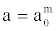and translation becomes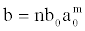where a0 and b0 are the discrete steps of the scale and translation, respectively . Then the wavelet can be represented by: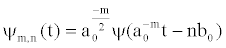(1)

The discrete wavelet transform is given by: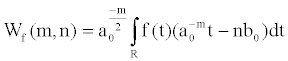(2)

Where,,mnZ∈, and Z is the set of integer numbers.

The parameter m which is called level, determines the wavelet frequency, while the parameter n indicates its position. The inverse discrete wavelet transform is given by: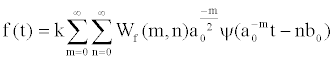(3)

Where, k is a constant that depends on the redundancy of the combination of the lattice with the used mother wavelet .

Along with the time-scale plane discretization, the independent variable (time) can also be discretized. The sequence of discrete points of the discretized signal can be represented by a Discrete Time Wavelet Series DTWS. The discrete time wavelet series is defined in relation to a discrete mother wavelet h(k). The discrete wavelet time series maps a discrete finite energy sequence to a discrete grid of coefficients. The discrete time wavelet series is given by .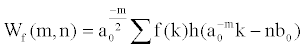(4)

Multi-Resolution Analysis (MRA)

Multi-resolution Analysis - MRA, aims to develop a signal f (t) representation in terms of an orthogonal basis which is composed by the scale and wavelet functions. An efficient algorithm for this representation was developed in 1988 by Mallat  considering a scale factor a0=2 and a translation factor b0=1. This means that at each decomposition level m, scales are a power of 2 and translations are proportional to powers of 2. Scaling by powers of 2 can be easily implemented by decimation (sub-sampling) and over-sampling of a discrete signal by a factor of 2. Sub-sampling by a factor of 2, involves taking a signal sample from every two available ones, resulting in a signal with half the number of samples than the original one. Over-sampling by a factor of 2, consists of inserting zeroes between each two samples resulting in a signal with twice the elements of the original one.

Analysis or decomposition

The structure of the multi-resolution analysis is shown in figure 2. The original signal passes through two filters, a low pass filter g (k), the function scale, and a high pass filter h (k), the mother wavelet. The impulse response of h (k) is related to the impulse response of g (k) by :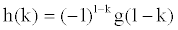(5)

Filter h (k), is the mirror of filter g (k), and they are called quadrature mirror filters.

In the structure presented in figure 2, the input signal is convolved with the impulse response of h (k), and g (k), obtaining two output signals. The low pass filter output represents the low frequency content of the input signal or an approximation of it. The high pass filter output represents the high frequency content of the input signal or a detail of it. It should be noted in figure 2 that the output provided by the filters has together twice the number of samples of the original signal.

This drawback is overcome by the process of decimation performed on each signal, thereby obtaining the signal CD, the wavelet coefficients that are the new signal representation in the wavelet domain, and the signal CA, the approximation coefficients which are used to feed the next stage of the decomposition process in an iterative manner resulting in a multi-level decomposition.

The decomposition process in figure 3 can be iterated with successive approximations being decomposed, then the signal being divided into several resolution levels. This scheme is called “wavelet decomposition tree” or “pyramidal structure” [12,14].

Figure 3 shows the schematic representation of a signal being decomposed at multiple levels.

Since the multi-resolution analysis process is iterative, it can theoretically be continued indefinitely. In fact, the decomposition can proceed only up to 1 (one) detail, consisting of a single sample. The maximum number of decomposition levels for a signal having N samples is given by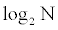Probabilistic Neural Network (PNN)

The structure of a Probabilistic Neural Network (PNN) is similar to a feed forward network. The main difference is that the activation function is no longer the sigmoid; it is replaced by a class of functions which includes, in particular, the exponential function. The main advantage of PNN is that it requires only one step for training and that the decision surfaces are close to the contours of the Bayes optimal decision when the number of training samples increases. Furthermore, the shape of the decision surface can be as complex as necessary, or as simple as desired .

The main drawback of PNN is that all samples used in the training process must be stored and used in the classification of new patterns. However, considering the use of high-density memories, problems with storage of training samples should not occur. In addition, the PNN processing speed in the classification of new patterns is quite satisfactory, and even several times faster than using back propagation algorithms as reported by .

The Bayes strategy for pattern classification: One of the traditionally accepted strategies or decision rules used in pattern classification is that they minimize the “expected risk” such strategies are called Bayes strategies, and can be applied to problems containing any number of categories .

To illustrate the Bayes decision rule formalism, it is considered the situation of two categories in which the state of known nature θ, can be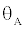or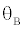It is desired to decide whether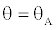or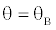based on a measurements set represented by n dimension vector x. Then the Bayes decision rule is given by: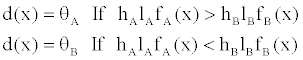(6)

Where fA(x) and fB(x) are the probability density functions for categoriesandrespectively, lA is the uncertainty function associated with the decision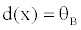when;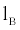is the uncertainty function associated with the decision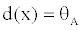when,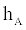is the a priori probability of categorypatterns occurrence, and hB=1-hA is the a priori probability thatThen, the boundary between the regions in which the Bayes decisionandis given by: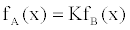(7)

Where: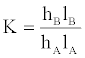(8)

It should be noted that, in general, the decision surfaces of two categories defined by equation 8 can be arbitrarily complex, since there are no restrictions on the densities except for those conditions to which all probability density functions must satisfy, namely that they must be always positive, and integer and their integrals over all space be equal to unity.

The ability to estimate the probability density functions, based on training patterns, is fundamental to the use of equation 8. Frequently, a priori probability can be known or estimated, and the loss functions require subjective evaluation. However, if the probability densities of the categories patterns to be separate are unknown, and all that is known is a set of training patterns, then, these patterns provide the only clue to the estimation of that unknown probability density. A particular estimator that can be used is :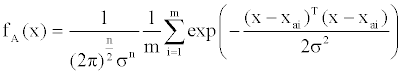(9)

Where i is the pattern number, m is the total number of training patterns, xai is the ith training pattern of categoryandis the smoothing factor. It should be noted that fA(x) is simply the sum of small Gaussian distributions centered at each training sample.

Structure of the probabilistic neural network: The probabilistic neural network is basically a Bayesian classifier implemented in parallel. The PNN, as described by Specht , is based on estimation of probability density functions for the various classes established by the training patterns. A schematic diagram for a PNN is shown in figure 4. The input layer X is responsible for connecting the input pattern to the radial basis layer. X= [X1, X2, X3,…..XM] is a matrix containing the vectors to be classified.

In the radial basis layer, the training vectors are stored in a weights matrixW1. When a new pattern is presented to the input, the block distance calculates the Euclidean distance between each input pattern vector for each of the stored weight vectors. The vector in the output block distance is multiplied, point by point, by the polarization factor b. The result of this multiplication n1 is applied to a radial basis function providing as output a1, obtained from: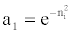(10)

This way, a vector in the input pattern close to a training vector is represented by a value close to 1 in the output vectora1. The competitive layer of the weight matrix w2 contains the target vectors representing each class corresponding to each vector in the training pattern. Each vector w2 has a1 only in the row associated with a particular class and 0 in other positions. The Multiplication w2a1 adds the a1 elements corresponding to each class, providing the output n2. Finally block C provides 1 at output a2 corresponding to the biggest element of n2 and 0 for the other values. Thus, the neural network classifies each vector of the input pattern in a specific class, because that class has the highest probability of being correct. The main advantage of PNN is its easy and straightforward project, and not depending on training.

#### Feature Extraction

Wavelet-feature extraction

Feature extraction is a pre-processing operation that transforms a pattern from its original form to a new form suitable for further processing. The signals acquired in time domain can be used to perform fault diagnosis. Discrete wavelet transform (DWT) has been widely used and provides the physical characteristics of time-frequency domain data. Wavelet analysis of vibration signals yields different descriptive parameters. A big set of parameters were selected as the basis for the study. These features were extracted from vibration signals.

In this paper, DWT of different versions of different wavelet families have been considered. The list of families considered for this study is given below:

1. Daubechies wavelet (db1, db2, db3, db4, db5, db6, db7, db8, db9, db10).

2. Coiflet (coif1, coif2, coif3, coif4, coif5)

3. Bi-orthogonal wavelet (bior1.1, bior1.3, bior1.5, bior2.2, bior2.4, bior2.6, bior2.8, bior3.5, bior3.7, bior3.9, bior4.4, bior5.5, bior6.8).

4. Reversed Bi-orthogonal wavelet (rbio1.1, rbio1.3, rbio1.5, rbio2.2, rbio2.4, rbio2.6, rbio2.8, rbio3.1, rbio3.3, rbio3.5, rbio3.7, rbio3.9, rbio4.4, rbio5.5, rbio6.8)

5. Symlets (sym2, sym3, sym4, sym5, sym6, sym7, sym8).

6. Meyer wavelet.

The DWT of unevenness signals were computed for different conditions of the yarn. They show a combination of signals and their decomposition details at different levels designated ‘d1’ to ‘d5’. Actually, for the analysis here, six levels are considered (from ‘d1’ to ‘d6’).

Feature definition

The proper processing of the signal shows that there are considerable changes in the average energy level for different conditions of the yarn. Feature extraction constitutes computation of specific measures, which characterize the signal. The Discrete Wavelet Transform (DWT) provides an effective method for generating features.

The collection of all such features forms the feature vector. A feature vector is given by: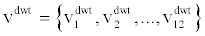A component in the feature vector is related to the individual resolutions by the following equation: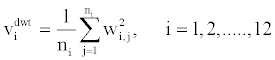Where,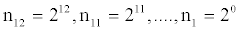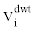is the ith feature element in a DWT feature vector. ni is the number of samples in an w2i,j individual sub-band, is the jth detail coefficient (high frequency component) of the ith sub-band. The wavelets considered in the present investigation are Haar (db1), Daubechies, Symlets, Coiflets, Biorthogonal, Reverse Biorthogonal and Meyer (dmey). Each of them is considered in the DWT form.

#### Classification

Naive Bayesian Classifier

The naive Bayesian classifier works as follows:

1. Let T be a training set of samples, each with their class labels. There are k classes C1, C2, C3….CK. Each sample is represented by an n-dimensional vector, X=X1,X2,….. XM, depicting measured values of the n attributes, A1, A2,….An respectively.

2. Given a sample X, the classifier will predict that X belongs to the class having the highest a posteriori probability, conditioned on X. That X is predicted to belong to the class Ci if and only if: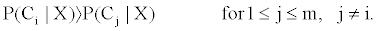Thus we find the class that maximizes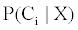The class Ci for whichis maximized is called the maximum posteriori hypothesis. By Bayes theorem: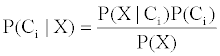3. As P(X) is the same for all classes, only P(X/ Ci) P (Ci) need be maximized. If the class a priori probability, P(Ci) is not known, then it is commonly assumed that the classes are equally likely, that is, P(C1)= P(C2)=…=P(Ck) and we would therefore maximize. P(X/Ci) Otherwise we maximize P(X/Ci) P (Ci). Note that the class a priori probability may be estimated by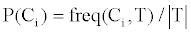4. A given data sets with many attributes; it would be computationally expensive to compute P(X/Ci). In order to reduce computation in evaluating P(X/Ci)P(Ci) the naive assumption of class conditional independence is made. This presumes that the values of the attributes are conditionally independent of one another, given the class label of the sample. Mathematically this means that: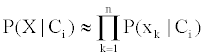The probabilities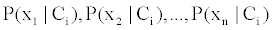can easily be estimated from the training set. Recall that here xk refers to the value of attribute Ak for sample X.

(a) If Ak is categorical, then P (xk/Ci) is the number of samples of class Ci in T having the value xk for attribute Ak, divided by freq (Ci, T), the number of sample of class Ci in T.

(b) If Ak is continuous-valued, then we typically assume that the values have a Gaussian distribution with a mean μ and standard deviation σ defined by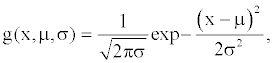So that: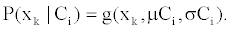We need to compute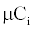and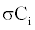which are the mean and standard deviation of values of attribute Ak for training samples of class Ci.

5. In order to predict the class label of X,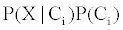is evaluated for each class Ci. The classifier predicts that the class label of X is Ci if and only if it is the class that maximizesBayesian network

A Bayesian Network (BN)  is a probabilistic graphical model where each variable is a node. The edges of the graph represent dependencies between linked nodes. A formal definition of Bayesian network  is a couple {G, P} where:

{G} is a directed acyclic graph, whose nodes are random variables X={X1, X2, X3,…….Xn}and whose missing edges represent conditional independences between the variables,

{P} is a set of conditional probability distributions (one for each variable):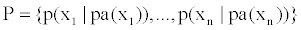Where pa(xi) is the set of parents of the node Xi.

The set P defines the joint probability distribution as: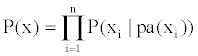Theoretically, variables X1, X2, X3,…….Xn can be discrete or continuous. But, in practice, for exact computation, only the discrete and the Gaussian case can be treated. Such a network is often called Conditional Gaussian Network (CGN).

In this context, to ensure availability of exact computation methods, discrete variables are not allowed to have continuous parents [20,21].

Practically, the conditional probability distribution is described for each node by his Conditional Probability Table (CPT). In a CGN, three cases of CPT can be found. The first one is for a discrete variable with discrete parents. By example, we take the case of two discrete variables A and B of respective dimensions a and b (with a1, a2, a3,…….aa the different modalities of A, and b1, b2,b3,…….bn the different modalities of B). If A is the parent of B, then the CPT of B is represented in table 1.

 A B b1 b2 … bb a1 a2 . . . aa P(b1|a1) P(b1|a2) . . . P(b1|aa) P(b1|a1) P(b1|a2) . . . P(b1|aa) ... ... . . . … P(bb|a1) P(bb|a2) . . . P(bb|aa)

Table 1: CPT of a discrete node with discrete parents.

We can see that the utility of the CPT is to condense the information about the relations of B with his parents. We can denote that the dimension of this CPT is a×b. In general the dimension of the CPT of a discrete node (dimension x) with p parents (discrete) Y1, Y2, Y3,…….Yp (dimension Y1, Y2, Y3,…….Yp) is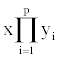The second case of CPT is for a continuous variable with discrete parents. Assuming that B is a Gaussian variable, and that A is a discrete parent of B with a modality, the CPT of B can be represented as in the table 2 where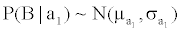indicates that B conditioned to A=ai follows a multivariate normal density function with parameters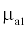and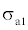If we have more than one discrete parent, the CPT of B will be composed of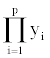Gaussian distribution where yi represents the respective number of modalities of the parent nodes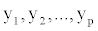A B a1a2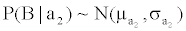. . . aa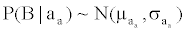Table 2: CPT of a Gaussian node with discrete parents.

The third case is when a continuous node B has a continuous parent A. In this case, we obtain a linear regression and we can write, for a fixed value a of A, that B follows a Gaussian distribution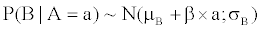where β is the regression coefficient. Evidently, the three different cases of CPT enumerated can be combined for different cases where a continuous variable has several discrete parents and several continuous (Gaussian) parents.

The classical use of a Bayesian network (or Conditional Gaussian Network) is to enter evidence in the network (evidence is the observation of the values of a set of variables). Thus, the information given by the evidence is propagated on the network in order to update the knowledge and obtain a posteriori probability on the non-observed variables. This propagation mechanism is called inference. As its name suggests, in a Bayesian network, the inference is based on the Bayes rule. Many inference algorithms (exact or approximate) have been developed, but one of the more exploited is the junction tree algorithm .

#### Application and Results

Database

To investigate the effectiveness of exact wavelet analysis in industrial machine fault diagnosis, a series of unevenness yarn signals collected from a real machine were analyzed for detecting possible faults occurring during the operation of the machine.

In the experiments, the type of fault is extremely frequent not only in the products prior to spinning but also in yarns, because defective card clothing, out of center running rollers in draw boxes, defective aprons, etc. can all produce periodic mass variations. It is unfortunately not possible in most cases that one can recognize and analyze this type of fault from the diagram.

In this study, 250 samples (25 bobbin x10 reading/bobbin) have been used for cotton yarn spinning Ne=30 carded at different times. The geometric parameters of the yarn irregularity are listed in chart 1.

Experiential work

The features were extracted from yarn diagram signals by DWT. Figure 5 shows the comparison of a signal under normal conditions and some yarn fault.

DWT of different versions of different wavelet families have been considered. The DWT of yarn signals were computed for different conditions of the yarn. Table 3 shows the decomposition details at different levels designated ‘d1’ to ‘d8’.

 Sample No. DWT Family CD1 CD2 CD3 CD4 CD5 CD6 CD7 CD8 CD9 CD10 CD11 CD12 CD13 CD14 CD15 CA16 1 Daubechies wavelet db1 99.997 0.0001783 0.00022197 0.00022399 0.00019189 0.00026164 0.00018585 0.0002606 0.0002546 0.00020664 0.00019651 0.00018787 0.00017379 0.00017567 0.00022152 0.00017723 db2 99.997 0.00026045 0.00016465 0.00022747 0.00018453 0.00028336 0.00022444 0.00018293 0.0003126 0.0001836 0.00016666 0.00020986 0.00019422 0.00023475 0.00020495 0.00017124 . . . . . . . . . . . . . . . . . . . . . . . . . . . . . . . . . . . . . . . . . . . . . . . . . . . db8 99.997 0.00022263 0.00018638 0.00020046 0.00020037 0.00019609 0.00019762 0.00031869 0.00025198 0.00022696 0.00018771 0.00021457 0.00015961 0.00024812 0.00016508 0.00016055 Symlets sym2 99.997 0.00026045 0.00016465 0.00022747 0.00018453 0.00028336 0.00022444 0.00018293 0.0003126 0.0001836 0.00016666 0.00020986 0.00019422 0.00023475 0.00020495 0.00017124 sym3 99.997 0.00020799 0.00020346 0.00022202 0.00018642 0.00025273 0.00019362 0.00019837 0.000284 0.0001843 0.00016106 0.00018058 0.00020675 0.00021117 0.00020348 0.00022653 . . . . . . . . . . . . . . . . . . . . . . . . . . . . . . . . . . . . . . . . . . . . . . . . . . . sym8 99.997 0.00018214 0.00019376 0.00019823 0.00022481 0.00020146 0.00019353 0.00026014 0.00023019 0.00020619 0.00015782 0.00027418 0.00020571 0.00019459 0.00017708 0.0001699 Coiflet coif1 99.997 0.00021123 0.00021147 0.00018553 0.00019129 0.00021946 0.00016608 0.0002942 0.00029378 0.0002052 0.00018334 0.00014875 0.00016665 0.00023394 0.00019063 0.00021046 coif2 99.997 0.00018383 0.00019875 0.00021838 0.00018553 0.00023329 0.00018571 0.00027301 0.00025832 0.00018341 0.00015788 0.00034107 0.00016806 0.00020297 0.00017774 0.00016242 . . . . . . . . . . . . . . . . . . . . . . . . . . . . . . . . . . . . . . . . . . . . . . . . . . . coif5 99.997 0.00026069 0.0001934 0.00015844 0.00016932 0.00022627 0.00021382 0.00025017 0.00027601 0.00018925 0.0001515 0.00031717 0.00020138 0.00019988 0.00017114 0.00016127 Bi-orthogonal boir1.1 99.997 0.0001783 0.00022197 0.00022399 0.00019189 0.00026164 0.00018585 0.0002606 0.0002546 0.00020664 0.00019651 0.00018787 0.00017379 0.00017567 0.00022152 0.00017723 boir1.3 99.997 0.00018179 0.00019912 0.00026472 0.00023137 0.00018622 0.00022144 0.00028852 0.0002788 0.00020255 0.00017273 0.00024966 0.0001799 0.0002345 0.00017455 0.00015931 . . . . . . . . . . . . . . . . . . . . . . . . . . . . . . . . . . . . . . . . . . . . . . . . . . . boir6.8 99.997 0.00028865 0.00023732 0.00016249 0.00031448 0.0002484 0.00017123 0.00020884 0.00026679 0.00018253 0.00029194 0.00022546 0.0001687 0.00015074 0.00015132 0.00013046 2 3 . . . . . . . . . . . . . . . . . . . . . . . . . . . . . . . . . . . . . . . . . . . . . . . . . . . . . . . . . 250 boir6.8 99.991 0.00098693 0.0013716 0.00011586 0.0026709 0.0010462 0.00025606 5.07E-05 0.0007915 0.00050491 0.00062304 0.00010003 0.00011233 8.03E-05 4.45E-05 1.01E-05

Table 3: Decomposition details of yarn signal.

From the calculated wavelet features the classification was carried out using Naïve Bayes and Bayes net classifiers and the results were compared. To design the classifiers (Naïve Bayes and Bayes net) for better classification, it has to satisfy two contradicting objectives.

During the training process, the algorithm gets trained in such a way that it performs well for the future data samples as shown in figure 6. As a first step the classification accuracy is found using Naïve Bayes and Bayes net classifiers for different versions of the wavelet family. In the similar fashion, the efficiencies of different versions of all the mentioned wavelet families were computed and plotted as histogram charts as shown in figure 7.

From figures 8-11, best version of different families was picked up from each of the charts and compared among those best versions of different wavelet families and found the overall best wavelet family and the best version of that family for that particular classifier. There were two challenges to be encountered to select the best one. First is to select the best classifier between the Naïve Bayes and Bayes net. Second is to select the best wavelet and its corresponding version. By visual inspection, one can understand that Bayes net classifier performs relatively better than the Naïve Bayes classifier. Hence, the first challenge can be easily overcome. In that particular classifier, the best wavelet was to be found. While comparing the performances of different wavelet families, dwt_db8 (99.87%), dwt_sym5 (100%), dwt_bior5.5 (99.84%), and dwt_coif (100%) were selected from figures 12-13 respectively as the best versions of different wavelet families. The confusion comes in picking up the best when two versions of the wavelet family give the same classification accuracies.

All the best versions of different wavelet families were compared and overall best wavelet and the wavelet family were found and plotted as a histogram chart as shown in figure 12.

From figure 13, one can clearly say that the best wavelets from the chart are dwt_sym3 and dwt_coif2 and the classification accuracy achieved is 100%. However, this performance of the different versions of the wavelet is for the specific conditions of the yarn mass variation as discussed. The result of the best versions of the wavelets can be illustrated in a better way using the confusion matrix as shown in figure 13.

From the confusion matrix (Figure 13), one can understand that 250 samples were considered for each condition of the yarn mass variation. All the diagonal elements of the confusion matrix represent the number of correctly classified data points and the non-diagonal elements represent the incorrectly classified data points. In this fashion, the classification accuracies were found and compared for various types of wavelets of different families. In this case, all the better condition data points have been correctly classified and the same was the case with drafting Front roller Fault (FrF) data points and fault with both Medial roller Fault (MrF) and Back Roller Fault (BrF). As there were no misclassification and hence efficiency was calculated to be 100%. The results obtained were specific to this particular data set. Classification accuracy of 100% does not assure similar performance for all feature datasets. In general the classification accuracy was very high. Hence the dwt_sym3 and dwt_coif2 versions of the wavelets are very much suited for fault diagnosis of yarn mass variation.

#### Conclusion

In this paper, an automatic yarn fault classification technique based on Multi-Resolution Analysis (MRA) and probabilistic neural networks has been developed. Five classical states viz., Without Fault (WOF), drafting Front roller Fault (FrF), drafting Medial roller Fault (MrF), drafting Back roller Fault (BrF), and Drafting Wave Fault (DWF), were simulated on yarn mass variation. Set of features was extracted using different wavelet analysis of data provided by a tested sample.

The probabilistic neural network PNN can be used to classify the yarn unevenness signals in a very incipient stage with a success rate of up to 90%. Naïve Bayes and Bayes net algorithms are used to classify and the results were compared in the form of histogram charts.

One can clearly say that, the feature extraction using wavelets as well as Bayes net algorithm for classification were found to be good candidates for practical applications of fault diagnosis of yarn mass variation. However, these results are calculated and presented only for the representative data points considered for the faulty conditions.

#### References

Select your language of interest to view the total content in your interested language

### Article Usage

• Total views: 12974
• [From(publication date):
April-2013 - Mar 27, 2019]
• Breakdown by view type
• HTML page views : 9157

## Post your commentCan't read the image? click here to refresh
###### Peer Reviewed Journals

Make the best use of Scientific Research and information from our 700 + peer reviewed, Open Access Journals

International Conferences 2019-20

Meet Inspiring Speakers and Experts at our 3000+ Global Annual Meetings

Top